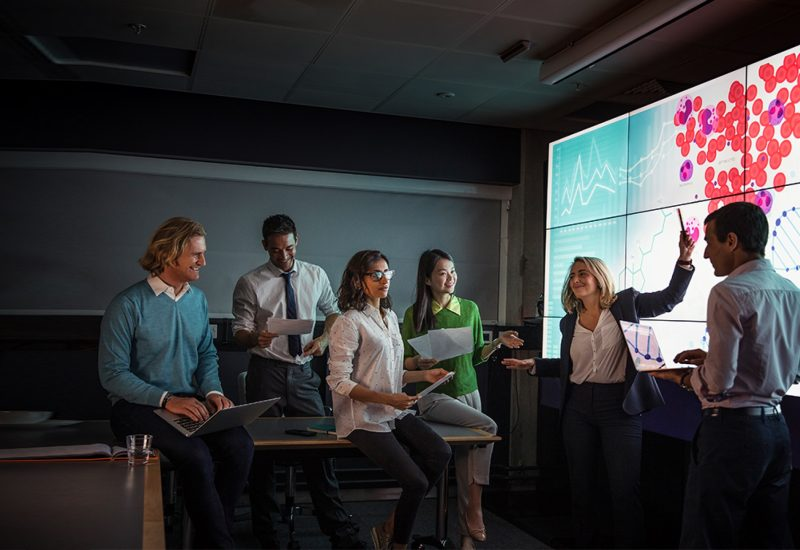Charles River Lecture Series

Charles River Lectures on Probability and Related Topics

Jointly organized by Harvard University, Massachusetts Institute of Technology, and Microsoft Research New England, the Charles River Lectures on Probability and Related Topics is a one-day event for the benefit of the greater Boston area mathematics community.

Committee Members

Alexei Borodin
Henry Cohn
Elchanan Mossel
Philippe Rigollet
Scott Sheffield
Nike Sun
Horng-Tzer Yau

Agenda

Friday, October 12, 2018

 Time Speaker Title 9:00 AM-9:30 AM Registration 9:30 AM-10:30 AM Eyal Lubetzky Dynamics for 2D Potts/FK models at criticality: many questions and a few answers 10:45 AM-11:45 AM Peter Winkler Random Permutations and the Displacement Ratio 12:00 PM-1:30 PM Lunch Break 1:30 PM-2:30 PM Sandra Cerrai Large-time asymptotics in the Smoluchowski-Kramers approximation of infinite dimensional systems 2:45 PM-3:45 PM Joe Neeman The Gaussian double-bubble 3:45 PM-4:30 PM Break 4:30 PM-5:30 PM Vadim Gorin Macroscopic fluctuations through Schur generating functions

Abstracts

Dynamics for 2D Potts/FK models at criticality: many questions and a few answers

Eyal Lubetzky

We will survey recent developments and several of the many problems that remain open on the rate of convergence to equilibrium of Glauber dynamics for the Potts and FK model in Z^2 at criticality.

Random Permutations and the Displacement Ratio

Peter Winkler

The “displacement ratio” R is the ratio of two natural measures of how close a permutation is to the identity, and is known always to be between 1 and 2. We want to know:  what is R for a random permutation in S_n with a given  number m(n) of inversions?   The behavior of R as m(n) changes is not entirely proven but seems rather surprising.

Joint work with David Bevan, U. of Strathclyde.

Large-time asymptotics in the Smoluchowski-Kramers approximation of infinite dimensional systems

Sandra Cerrai

I will discuss the validity of the so-called Smoluchowski-Kramers approximation for systems with an infinite number of degrees of freedom in a finite time. Then, I will investigate the validity of such approximation for large time. In particular, I will address the problem of the convergence, in the small mass limit, of statistically invariant states for a class of semi-linear damped wave equations, perturbed by an additive Gaussian noise, with quite general nonlinearities. More precisely, I will show how the first marginals of any sequence of invariant measures for the stochastic wave equation converge in a suitable Wasserstein metric to the unique invariant measure of the limiting stochastic semi-linear parabolic equation obtained in the Smoluchowski-Kramers approximation.

The Gaussian double-bubble

Joe Neeman

The Gaussian isoperimetric inequality states that if we want to partition R^n into two sets with prescribed Gaussian measure while minimizing the Gaussian surface area of the interface between the sets, then the optimal partition is obtained by cutting R^n with a hyperplane. We prove an extension to more than two parts. For example, the optimal way to partition R^3 into three parts involves cutting along three rays that meet at 120-degree angles at a common point. This is the Gaussian analogue of the famous Double Bubble Theorem in Euclidean space, which describes the shape that results from blowing two soap bubbles and letting them stick together.

Joint work with Emanuel Milman.